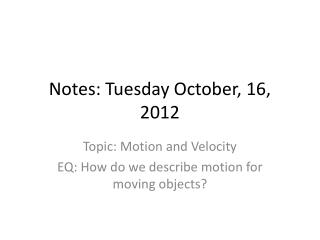DownloadDownload PresentationNotes: Tuesday October, 16, 2012

# Notes: Tuesday October, 16, 2012

Télécharger la présentation## Notes: Tuesday October, 16, 2012

- - - - - - - - - - - - - - - - - - - - - - - - - - - E N D - - - - - - - - - - - - - - - - - - - - - - - - - - -
##### Presentation Transcript

1. Notes: Tuesday October, 16, 2012 Topic: Motion and Velocity EQ: How do we describe motion for moving objects?

2. 4.1Motion Is Relative An object is moving if its position relative to a fixed point is changing.

3. 4.1Motion Is Relative • Even things that appear to be at rest move. • When we describe the motion of one object with respect to another, we say that the object is moving relative to the other object. • A book that is at rest, relative to the table it lies on, is moving at about 30 kilometers per second relative to the sun. • The book moves even faster relative to the center of our galaxy.

4. 4.1Motion Is Relative • When we discuss the motion of something, we describe its motion relative to something else. • The space shuttle moves at 8 kilometers per second relative to Earth below. • A racing car in the Indy 500 reaches a speed of 300 kilometers per hour relative to the track. • Unless stated otherwise, the speeds of things in our environment are measured relative to the surface of Earth.

5. 4.1Motion Is Relative Although you may be at rest relative to Earth’s surface, you’re moving about 107,000 km/h relative to the sun.

6. Try Problems 1 and 2 on page 62 Write the answers on your notes

7. 4.1Motion Is Relative How can you tell if an object is moving?

8. 4.2Speed You can calculate the speed of an object by dividing the distance covered by time.

9. 4.2Speed Before the time of Galileo, people described moving things as simply “slow” or “fast.” Such descriptions were vague. Galileo is credited as being the first to measure speed by considering the distance covered and the time it takes. Speed is how fast an object is moving.

10. 4.2Speed • Any combination of units for distance and time that are useful and convenient are legitimate for describing speed: • miles per hour (mi/h) • kilometers per hour (km/h) • centimeters per day (cm/d) • light-years per century (ly/cn)

11. 4.2Speed We will primarily use the unit meters per second (m/s) for speed. If a cheetah covers 50 meters in a time of 2 seconds, its speed is 25 m/s.

12. 4.2Speed

13. 4.2Speed Instantaneous Speed A car does not always move at the same speed. You can tell the speed of the car at any instant by looking at the car’s speedometer. The speed at any instant is called the instantaneous speed.

14. 4.2Speed Average Speed In a trip by car, the car will certainly not travel at the same speed all during the trip. The driver cares about the average speed for the trip as a whole. The average speed is the total distance covered divided by the time.

15. 4.2Speed Average speed can be calculated easily: For example, a distance of 240 kilometers during a time of 4 hours is an average speed of 60 km/h:

16. 4.2Speed If we know average speed and travel time, the distance traveled is easy to find. total distance covered = average speed × travel time For example, if your average speed is 80 kilometers per hour on a 4-hour trip, then you cover a total distance of 320 kilometers.

17. 4.2Speed think! If a cheetah can maintain a constant speed of 25 m/s, it will cover 25 meters every second. At this rate, how far will it travel in 10 seconds? In 1 minute?

18. 4.2Speed think! If a cheetah can maintain a constant speed of 25 m/s, it will cover 25 meters every second. At this rate, how far will it travel in 10 seconds? In 1 minute? Answer:In 10 s the cheetah will cover 250 m, and in 1 min (or 60 s) it will cover 1500 m.

19. 4.2Speed think! The speedometer in every car also has an odometer that records the distance traveled. If the odometer reads zero at the beginning of a trip and 35 km a half hour later, what is the average speed?

20. 4.2Speed think! The speedometer in every car also has an odometer that records the distance traveled. If the odometer reads zero at the beginning of a trip and 35 km a half hour later, what is the average speed? Answer:

21. 4.2Speed How can you calculate speed?

22. Try problems 3 and 4 on page 62 • And 26-31 on page 64